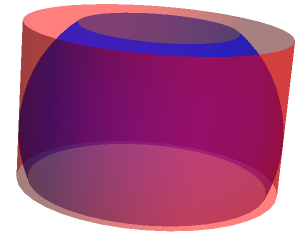# (W^2 + X^2) / (W^2 + X^2 + Y^2 + Z^2)

The Box-Müller transform is a method of transforming pairs of independent uniform distributions to pairs of independent standard Gaussians. Specifically, if U and V are independent uniform [0, 1], then define the following:

• ρ = sqrt(–2 log(U))
• θ = 2π V
• X = ρ cos(θ)
• Y = ρ sin(θ)

Then it follows that X and Y are independent standard Gaussian distributions. On a computer, where independent uniform distributions are easy to sample (using a pseudo-random number generator), this enables one to produce Gaussian samples.

As the joint probability density function of a pair of independent uniform distributions is shaped like a box, it is thus entirely reasonable to coin the term ‘Müller’ to refer to the shape of the joint probability density function of a pair of independent standard Gaussians.

### What about the reverse direction?

It transpires that it’s even easier to manufacture a uniform distribution from a collection of independent standard Gaussian distributions. In particular, if W, X, Y, and Z are independent standard Gaussians, then we can produce a uniform distribution using a rational function:$U := \dfrac{W^2 + X^2}{W^2 + X^2 + Y^2 + Z^2}$

The boring way to prove this is to note that this is the ratio of an exponential distribution over the sum of itself and another independent identically-distributed exponential distribution. But is there a deeper reason? Observing that the function is homogeneous of degree 0, it is equivalent to the following claim:

Take a random point on the unit sphere in 4-dimensional space (according to its Haar measure), and orthogonally project onto a 2-dimensional linear subspace. Then the squared length of the projection is uniformly distributed in the interval [0, 1].

This has a very natural interpretation in quantum theory (which seems to be a special case of a theorem by Bill Wootters, according to this article by Scott Aaronson arguing why quantum theory is more elegant over the complex numbers as opposed to the reals or quaternions):

Take a random qubit. The probability p of measuring zero in the computational basis is uniformly distributed in the interval [0, 1].

Discarding the irrelevant phase factor, qubits can be viewed as elements of S² rather than S³. (This quotient map is the Hopf fibration, whose discrete analogues we discussed earlier). Here’s a picture of the Bloch sphere, taken from my 2014 essay on quantum computation:

Then, the observation reduces to the following result first proved by Archimedes:

Take a random point on the unit sphere (in 3-dimensional space). Its z-coordinate is uniformly distributed.

Equivalently, if you take any slice containing a sphere and its bounding cylinder, the areas of the curved surfaces agree precisely:There are certainly more applications of Archimedes’ theorem on the 2-sphere, such as the problem mentioned at the beginning of Poncelet’s Porism: the Socratic Dialogue. But what about the statement involving the 3-sphere (i.e. the preimage of Archimedes’ theorem under the Hopf fibration), or the construction of a uniform distribution from four independent standard Gaussians?

This entry was posted in Uncategorized. Bookmark the permalink.

### 2 Responses to (W^2 + X^2) / (W^2 + X^2 + Y^2 + Z^2)

1.Warren D Smith says:

So if we have two i.i.d.. gaussian randoms, call them X and Y:
1. divide their sum of squares S by a random uniform(0,1);
2. subtract S; result is T;
3. generate a random unit-length 2-vector then rescale to length sqrt(T).
This is a way to generate two new i.i.d. gaussian randoms from two old ones, plus two uniform
randoms. The algorithm (1+2+3) clearly is more efficient than Box-Muller in the sense no logs are needed. Only divisions and square roots are needed.

2.Warren D Smith says:

Also I think your whole result here is not new in the sense I saw something like it (if not identical) before, not sure where…